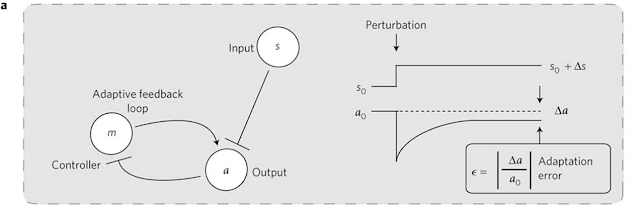#77 Brownian Carnot engine-I 微觀卡諾引擎-I

After the discussion of detailed balance, let's back to our adaptive sensory system again:Figure source: Nat Phys 8:422–428. Fig. 1a. A schematic model of sensory adaptation system

The dynamics of this system could be modeled by a coupled Langevin equations:
in which η represents our old friend -- white noise, which means

The functionality of Fa & Fm

Now let's consider the functionality of Fa & Fm. What should they look like? Since this is a system with adaptation, given a fixed output s and controller m, there should have a steady state output value of a given certain & m. Let's define such steady state output value as G(s,m). According to the basic setting of the system, we know that:
If we fixed s & m, the output value a has to relax to this steady state output value. So the governing equation of a is now clear:

What about m? This is a little bit complex. The authors of this research hope that we could discuss both an equilibrium system and a nonequilibrium system in the same equation, so they hope the governing equation of m could look like this:

So the problem becomes how to express the equilibrium and nonequilibrium terms and combine them back together. The equilibrium term is easy since we have obtained the requirement set by detailed balance in our previous episode:
Plug in the Fa we have just obtained and we will have

This means if the system satisfy detailed balance, the system will always return to (a0, m0) no matter how the external signal changes. Such system will have no adaptation at all.

What about the nonequilibrium term? Since the negative feedback loop will require m to decrease as a increases, one simple form would be:
Combine these 2 terms together and we will get our final functionality of Fm:

Linear Stability Analysis

Now we have our coupled Langevin equations:
Is there any fixed point in this system? If any, is it stable? To simplify our analysis, we let
There is one fixed point (a*, m*) that makes a* = a0, G(s, m*) = a0. To determine its stability, we write down the Jacobian matrix:
The determinant and the 1/4(trJ)^2 are
Since the trace of the Jacobian < 0, if the determinant of the Jacobian is greater than 0, the fixed point would be either a stable node or a stable spiral. However, if the determinant is lesser than 0, the fixed point would be a saddle point.